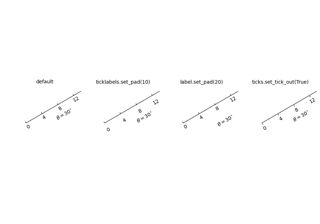# mpl_toolkits.axisartist.grid_finder.ExtremeFinderSimple#

class mpl_toolkits.axisartist.grid_finder.ExtremeFinderSimple(nx, ny)[source]#

Bases: `object`

A helper class to figure out the range of grid lines that need to be drawn.

Parameters:
nx, nyint

The number of samples in each direction.

__call__(transform_xy, x1, y1, x2, y2)[source]#

Compute an approximation of the bounding box obtained by applying transform_xy to the box delimited by `(x1, y1, x2, y2)`.

The intended use is to have `(x1, y1, x2, y2)` in axes coordinates, and have transform_xy be the transform from axes coordinates to data coordinates; this method then returns the range of data coordinates that span the actual axes.

The computation is done by sampling `nx * ny` equispaced points in the `(x1, y1, x2, y2)` box and finding the resulting points with extremal coordinates; then adding some padding to take into account the finite sampling.

As each sampling step covers a relative range of 1/nx or 1/ny, the padding is computed by expanding the span covered by the extremal coordinates by these fractions.

## Examples using `mpl_toolkits.axisartist.grid_finder.ExtremeFinderSimple`#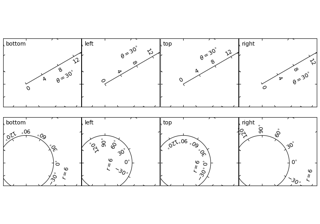axis_direction demo

axis_direction demo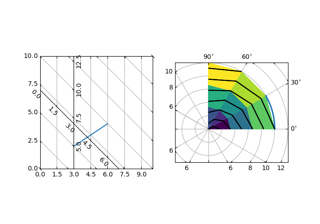Curvilinear grid demo

Curvilinear grid demo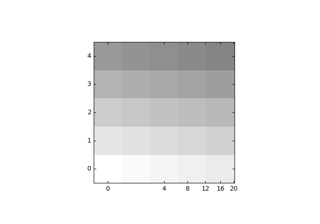Demo CurveLinear Grid2

Demo CurveLinear Grid2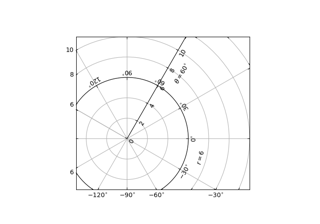floating_axis demo

floating_axis demo